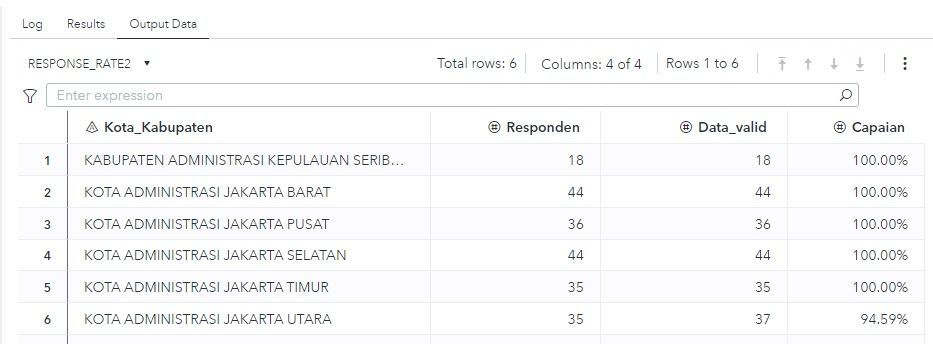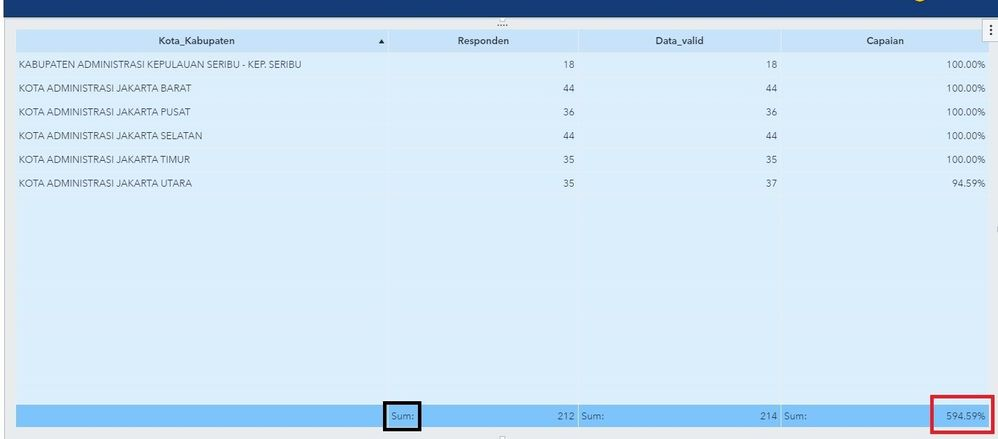## Percentage total in List Table in SAS Visual Analytics wrong

Hello everyone.

I am using SAS code to get data from API, and I want to create a table that shown response rate. To create that table, I am using this code:

``````proc sort data=work.TARGET_SKLM_KOTAKAB3;by KotaKabKecKel;run;
proc sort data=work.RESPONDEN_SKLM_KOTKAB2;by KotaKabKecKel;run;
data work.Response_Rate;
length KotaKabKecKel \$200;
merge work.RESPONDEN_SKLM_KOTKAB2(in=a) work.TARGET_SKLM_KOTAKAB3(in=b);
by KotaKabKecKel;
if b;
format Capaian percent9.2;
Capaian	= Responden/Data_valid;
Kota_Kabupaten = KotaKabKecKel;
drop KotaKabKecKel;
run;

data Response_Rate2;
retain Kota_Kabupaten;
set Response_Rate;
run;``````

The result from that would look like this:Result from code

It's the right result, and then I promote the table into caslib so I can visualize it in Visual Analytics.

I then use List Table to show the result and it looks like this:List table

Because of default aggregation for measure data item is sum, the result looks like that.

What I want to ask, is there any way to rename "Sum:" in the total column?
And how to change the sum percentage to the right number ( 212/214 = 99.07% ) ?

1 ACCEPTED SOLUTION

Accepted Solutions

## Re: Percentage total in List Table in SAS Visual Analytics wrong

Yes you can achieve the same result using Proc REPORT, see code example below.

• I always use a computed variable (_dummy) as the last one in the column statement. This will allow me to access all values to the left of this column in the compute block. It has the NOPRINT option, so it is not printed
• Since the resp and valid columns are defined as ANALYSIS using SUM I can use the variable-name.statistic to access the value, this will also work for the report break line (RBREAK)
• In the compute block I can detect if I am in a break line and fill the variable kota accordingly

Proc REPORT will build the report line by line.

``````data have;
infile cards dlm=",";
input
kota : \$32.
resp : 8.
valid : 8.
;
cards;
seribu,18,18
barat,44,44
pusat,36,36
selatan,44,44
timur,35,35
utara,35,37
;

proc report data=have;
column kota resp valid capian _dummy;
define kota / group;
define resp / analysis sum;
define valid / analysis sum;
define capian / computed format=percent9.2;
define _dummy / computed noprint;

rbreak after / summarize style={backgroundcolor=lightblue};
compute _dummy ;
capian = resp.sum / valid.sum;
if _break_ = "_RBREAK_" then do;
kota = "Total";
end;
endcomp;
run;``````
4 REPLIES 4

## Re: Percentage total in List Table in SAS Visual Analytics wrong

Calculate the value for capaian within the report using an expression like this:

`Sum(_ByGroup_, resp) / Sum(_ByGroup_,valid)`

Change the format to percent, use your names for the resp and valid. This will give you the correct total value.

As for the text in the total line, there is currently not a way to change this. I would simply not display the labels for the total values.

## Re: Percentage total in List Table in SAS Visual Analytics wrong

Thanks for the answer. I've used that way before.

I thought that maybe I could get the same result like that in SAS Studio. Is there anyway to do that? Or it's not possible because when data promoted from SAS Studio, the data categorized as Measure Data with Sum as Aggregation?
Because when I compared between Capaian made from SAS Code and Capaian made with your way, the only difference is that the latter don't have Aggregation.

## Re: Percentage total in List Table in SAS Visual Analytics wrong

Yes you can achieve the same result using Proc REPORT, see code example below.

• I always use a computed variable (_dummy) as the last one in the column statement. This will allow me to access all values to the left of this column in the compute block. It has the NOPRINT option, so it is not printed
• Since the resp and valid columns are defined as ANALYSIS using SUM I can use the variable-name.statistic to access the value, this will also work for the report break line (RBREAK)
• In the compute block I can detect if I am in a break line and fill the variable kota accordingly

Proc REPORT will build the report line by line.

``````data have;
infile cards dlm=",";
input
kota : \$32.
resp : 8.
valid : 8.
;
cards;
seribu,18,18
barat,44,44
pusat,36,36
selatan,44,44
timur,35,35
utara,35,37
;

proc report data=have;
column kota resp valid capian _dummy;
define kota / group;
define resp / analysis sum;
define valid / analysis sum;
define capian / computed format=percent9.2;
define _dummy / computed noprint;

rbreak after / summarize style={backgroundcolor=lightblue};
compute _dummy ;
capian = resp.sum / valid.sum;
if _break_ = "_RBREAK_" then do;
kota = "Total";
end;
endcomp;
run;``````

## Re: Percentage total in List Table in SAS Visual Analytics wrong

That works. Thank you!
Discussion stats
• 4 replies
• 278 views
• 0 likes
• 2 in conversation